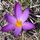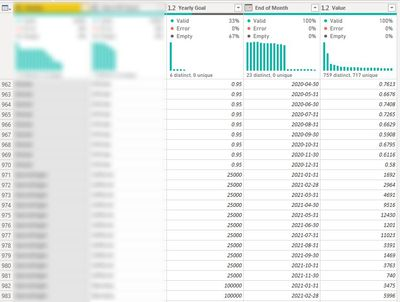cancel
Showing results for
Did you mean:Resolver I

## Unpivoting challenge (multiple Excel files with multiple spreadsheets from a Sharepoint folder)

Hi,

until now I manually managed to extract data from 2 Excel files located in a Sharepoint folder.

One file was related to 2020 data and the other to 2021 data.

Each Excel file contains 15 tabs with the same name and data structure. As displayed belowAt the end of all transformations this is the resultNow, I would like to automate my manual process for the years to come.

In other words, my goal would be to limit the update process of my dataset to 2 actions:

1. Copy the new data file into the Sharepoint folder

2. Refresh the Power BI dataset

And here is my challenge:

I just know how to read and filter the proper files from the Sharepoint folder. But after that, I have 2 problem to address:

a. how to iterate throughout all the Excel file Spreadsheets to read all data tables

b. how to unpivot my tables, when I have multiple years listed that should become my new date dimension, like in the example below

Situation before unpivoting (first spreadsheet of the 2 Excel files):Result expected:m

1 ACCEPTED SOLUTIONResolver I

Thank you @wdx223_Daniel ,

it does work, but the result is not exactly what I expected.

``````let
Source = SharePoint.Files("my Sharepoint path", [ApiVersion = 15]),
#"Filtered Rows" = Table.SelectRows(Source, each Text.StartsWith([Name], "my text filter")),
custom1=Table.Combine(List.TransformMany(#"Filtered Rows"[Content],each Excel.Workbook(_)[Data],(x,y)=>Table.UnpivotOtherColumns(y,{"Column1","Column2","Column3"},"End of Mounth","Value")))
in
custom1``````

and this is the resultAt the end I solved my need with the following solution

``````let
Source = SharePoint.Files("my Sharepoint url", [ApiVersion = 15]),
#"Filtered Rows" = Table.SelectRows(Source, each Text.StartsWith([Name], "my text filter")),

WorkbookList = List.Transform(#"Filtered Rows"[Content], each Excel.Workbook(_)[Data]),

TransformTable = (SpreadsheetList as list) as list =>
let
TransformedTable = List.Transform(
Table.UnpivotOtherColumns(
{"Col1", "Col2", "Col3"},
"Attribute", "Value"))
in
TransformedTable,

in

Thank you again @wdx223_Daniel for your answer, because helped me to understand better iterations in M, you deserve a Kudos!

m

2 REPLIES 2Super User

let

custom1=Table.Combine(List.TransformMany(source[Content],each Excel.Workbook(_)[Data],(x,y)=>Table.UnpivotOtherColumns(y,{the column name list which you do not want to unpivot},"End of Month","Value")))

in custom1Resolver I

Thank you @wdx223_Daniel ,

it does work, but the result is not exactly what I expected.

``````let
Source = SharePoint.Files("my Sharepoint path", [ApiVersion = 15]),
#"Filtered Rows" = Table.SelectRows(Source, each Text.StartsWith([Name], "my text filter")),
custom1=Table.Combine(List.TransformMany(#"Filtered Rows"[Content],each Excel.Workbook(_)[Data],(x,y)=>Table.UnpivotOtherColumns(y,{"Column1","Column2","Column3"},"End of Mounth","Value")))
in
custom1``````

and this is the resultAt the end I solved my need with the following solution

``````let
Source = SharePoint.Files("my Sharepoint url", [ApiVersion = 15]),
#"Filtered Rows" = Table.SelectRows(Source, each Text.StartsWith([Name], "my text filter")),

WorkbookList = List.Transform(#"Filtered Rows"[Content], each Excel.Workbook(_)[Data]),

TransformTable = (SpreadsheetList as list) as list =>
let
TransformedTable = List.Transform(
Table.UnpivotOtherColumns(
{"Col1", "Col2", "Col3"},
"Attribute", "Value"))
in
TransformedTable,

in

Thank you again @wdx223_Daniel for your answer, because helped me to understand better iterations in M, you deserve a Kudos!

m